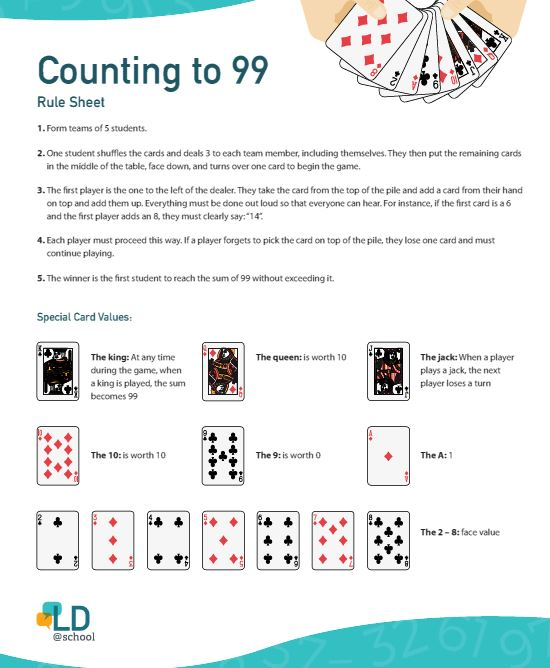By Nicole Lauzon

## Introduction"About 3 to 8% of school-aged children have a math learning disability."

Centre of Excellence for Early Childhood Development, 2011

Arithmetic must be integrated into classroom activities to allow students to acquire useful reflexes, not only at school, but also in their daily lives. It is an important part of mathematics, but more importantly, it is perhaps the most visible and the most "practiced" skill of all. In fact, this is why arithmetic and mathematics are often confused.

So what is arithmetic? It is the act of calculating and includes two fundamental aspects:

• choosing the operations and their proper sequence to obtain a desired result, such as creating an algorithm;
• executing those operations following immutable and precise rules such as applying an algorithm.

Only the first aspect requires the student to reflect and choose. The execution in itself leaves no latitude. In arithmetic activities unrelated to a problem, the manipulated symbols refer to nothing and come from nowhere. Nevertheless, some students accomplish them with ease. Thanks to their great memory, they apply the rules blindly, with few or no errors. Other students - and they are numerous - cannot do the proposed arithmetic operations without making many mistakes. In both cases, students do not know what they are doing or why they are doing it.

According to Chard & Gersten (1999), it is important for students to develop skills in number sense prior to their being able to solve basic arithmetic problems, as students must develop a sense of how numbers relate to each other before they begin performing even the most basic mathematical operations.

Children who have difficulty with arithmetic (dyscalculia or logico-mathematical disorders) need mathematics to be presented in a playful and concrete way. The use of manipulatives (sticks, macaroni, dice, decks of cards, coins, marbles, etc.) should be encouraged when teaching arithmetic operations; this may include the use of educational CD-ROMs, board games and online games to motivate students.

Explicit instruction has been shown to be extremely effective for students with learning disabilities. In order to apply this strategy to teaching arithmetic skills, this means that the educator must guide the student in applying a problem-solving approach by modeling the different steps. The use of visual devices is important because the child can use them at any time. This becomes an indispensable tool for the student. Moreover, it is important to allow the child to use this type of tool during assessments of all kinds in order that they are able to express their learning.

For example:

• Memorizing multiplication tables is difficult, so some students with math disabilities may require a chart grouping numbers into sets as an aid or they may need to use a calculator.
• Some students with math disabilities need more time to do mathematical problems and should be given extra time to do so.
• Priority must be given to teaching concepts; cognitive teaching based on diagrams and repetition exercises are important for students with difficulties or learning disabilities in mathematics to succeed.

Here is an interesting arithmetic activity called “99”. In this activity, which lasts approximately 30 minutes, students play cards and do mental arithmetic. Moreover, they must communicate appropriately, cooperate and use efficient methodologies. This activity is done in groups of five students.

Note: Generally, students whose logico-mathematical intelligence is well developed do mental arithmetic quickly, but other students, and not only those with learning disabilities, can find it more difficult.

Material:

• Sheets of paper
• Decks of cards

Steps:Feedback on the activity

Ask the students the following questions:

• Are you able to do mental arithmetic efficiently? Explain your answer.